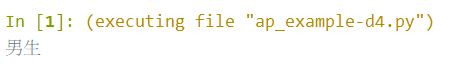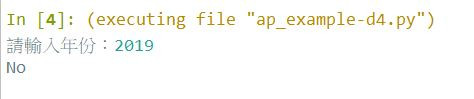#DAY 4
0

## 電腦的判斷基礎 -- If else 陳述句

``````sex = "male"

if(sex == "male"):
print("男生")
else:
print("不是男生")
``````### If Else 陳述句語法

``````if(判斷式1):
#如果判斷式1為真，執行此區
elif(判斷式2):
#如果判斷式2為真，執行此區
else:
#如果上述判斷式皆為假，執行此區
``````

### 比較運算子

##### 練習 4-1

``````0 < 5
7 >= 6
true != false
0 == 0
"true" == "true"
true = !false
1 = 1 #給我注意這一個!
``````

### 多重判斷敘述式

``````if(明天沒下雨 and 我有空):
去看電影()
``````

``````if(明天沒下雨 && 我有空):
去看電影()
``````

``````#你來我家找我或我去學校都可以拿東西給你
if(你到我家 or 我到學校):
拿東西給你()
``````

``````#你來我家找我或我去學校都可以拿東西給你
if(你到我家 || 我到學校):
拿東西給你()
``````

``````#你去參加聚餐我就不去
if(not(你參加聚餐)):
我參加()
``````
``````#你去參加聚餐我就不去
if(!你參加聚餐):
我參加()
``````

A and B A && B
A or B A || B
not(A) !A
##### 練習 4-2

``````(true || false) and false
0 and 1
not(0)
!false && true
!true or not(1)
``````

### 判斷應用，尋找親和數(Amicable Pair)

``````220 的所有因數有  1, 2, 4, 5, 10, 11, 20, 22, 44, 55, 110
284 = 1 + 2 + 4 + 5 + 10 + 11 + 20 + 22 + 44 + 55 + 110
284 的所有因數有  1, 2, 4, 71, 142
220 = 1 + 2 + 4 + 71 + 142
``````

``````#include<stdio.h>
#include<math.h>

int main(int argc, char *argv[])
{
long long limit;
long long start;

printf("Enter START END -->");
scanf("%lld %lld",&start,&limit);
printf("Program Start!\n");

for(long long number1 = start; number1<=limit ; number1++)
{
long long factor;

factor = 2;
long long totalA = 1;
long long number1_s = sqrt(number1);

while( factor < number1_s )
{
if( number1%factor == 0 )
{
//printf("%lld\n",factor);
totalA += factor;
totalA += number1/factor;
}

factor = factor+1;
}

factor = 2;
long long number2 = totalA;
long long totalB = 1;
long long number2_s = sqrt(number2);

while( factor < number2_s )
{
if( number2%factor == 0 )
{
totalB += factor;
totalB += number2/factor;
}

if(totalB > number1)
break;

factor = factor+1;
}

//printf("和 -> %d:%d",totalA,totalB);
if(totalA == number2 && totalB == number1 && number1 != number2)
{
printf("發現親和數 -> %lld:%lld\n",number1,number2);
}
}

return 0;
}
``````##### 練習 4-3

Tip:
<閏年規則>

• 每四年閏一年
• 逢百年不閏
• 逢四百年閏一年

``````year = 2019

year = int(input("請輸入年份：")) #取得使用者鍵盤輸入
if (year % 400 == 0):
print("Yes")   #是閏年

elif (year % 100 == 0):
print("No")    #不是閏年

elif (year % 4 == 0):
print("Yes")   #是閏年

else:
print("No")    #不是閏年
``````